### Home > A2C > Chapter Ch11 > Lesson 11.2.4 > Problem11-93

11-93.

1. Factor f(x) = x3 − 5x2 + 11x − 15.

2. Solve f(x) = 0.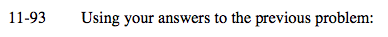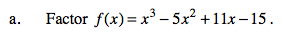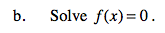Use a generic rectangle to see if x − 3 is a factor.

x2 − 2x + 5

(x − 3)(x2 − 2x + 5)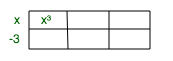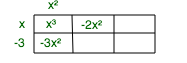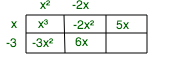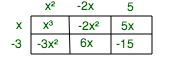Use the factors to solve for x.

(x − 3)(x2 − 2x + 5)

x = 3

Use the quadratic formula to solve the other factor.

$x=\frac{2\pm\sqrt{4-20}}{2}$

x = 1 ± 2i

x = {3, 1 ± 2i}